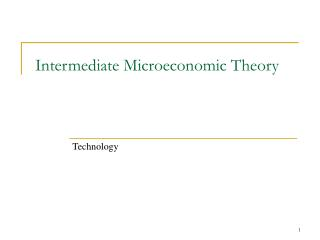Download PresentationIntermediate Microeconomic Theory

Intermediate Microeconomic Theory - PowerPoint PPT Presentation

Intermediate Microeconomic Theory. Technology. Inputs. In order to produce output, firms must employ inputs (or factors of production) Sometimes divided up into categories: Labor Capital Land . The Production Function.I am the owner, or an agent authorized to act on behalf of the owner, of the copyrighted work described.
Download PresentationIntermediate Microeconomic Theory

Download Policy: Content on the Website is provided to you AS IS for your information and personal use and may not be sold / licensed / shared on other websites without getting consent from its author.While downloading, if for some reason you are not able to download a presentation, the publisher may have deleted the file from their server.

- - - - - - - - - - - - - - - - - - - - - - - - - - E N D - - - - - - - - - - - - - - - - - - - - - - - - - -
Presentation Transcript
1. Intermediate Microeconomic Theory Technology

2. Inputs • In order to produce output, firms must employ inputs (or factors of production) • Sometimes divided up into categories: • Labor • Capital • Land

3. The Production Function • To produce any given amount of a good a firm can only use certain combinations of inputs. • Production Function – a function that characterizes how output depends on how many of each input are used. q = f(x1, x2, …, xn) units of output units of input 1 units of input 2…units of input n

4. Examples of Production Functions • What might be candidate production functions for the following? • Vodka Distillary – can be made from either potatoes or corn. • Axe Factory – each axe requires exactly one blade & one handle. • Car Wash – requires both Labor and “Capital”, though not necessarily in fixed proportions. • So what are Production functions analogous to? How are they different?

5. Production Functions vs. Utility Functions • Unlike in utility theory, the output that gets produced has cardinal properties, not just ordinal properties. • For example, consider the following two production functions: • f(x1,x2) = x10.5x20.5 • f(x1,x2) = x12x22

6. Isoquants • Isoquant – set of all possible input bundles that are sufficient to produce a given amount of output. • Isoquants for Vodka? • Isoquants for Axes? • Isoquants for Cars Washed? • So what are Isoquants somewhat analogous to? How do they differ?

7. Isoquants • Again, like with demand theory, we are most interested in understanding trade-offs. • What aspect of Isoquants tells us about trade-offs in the production process?

8. Marginal Product of an Input • Consider how much output changes due to a small change in one input (holding all other inputs constant), or • Now consider the change in output associated with a “very small” change in the input. • Marginal Product (of an input) – the rate-of-change in output associated with increasing one input (holding all other inputs constant), or

9. Marginal Product of an Input • Example: • Suppose you run a car wash business governed by the production function q = f(L, K) = L0.5K0.5 • (q = cars washed, L = Labor hrs, K = machine hrs.) • What will Isoquants look like? • What will be the Marginal Product of Labor at the input bundle {L=4, K= 9}? • What will be the Marginal Product of Labor at the input bundle {L=9, K= 9}?

10. Substitution between Inputs • Marginal Product is interesting on its own (unlike marginal utility) • MP also helpful for considering how to evaluate trade-offs in the production process. • Consider again the following thought exercise: • Suppose firm produces using some input combination (x1’,x2’). • If it used a little bit more x1, how much less of x2 would it have to use to keep output constant? x2 Δx1 x2’ x2” Δx2 f(x1”,x2’) f(x1’,x2’) x1’ x1” x1

11. Technical Rate of Substitution (TRS) • Technical Rate of Substitution (TRS): • TRS = Slope of Isoquant • Also referred to as Marginal Rate of Technical Substitution (MRTS) or Marginal Rate of Transformation (MRT) • So what would be the expression for the TRS for a generalized Cobb-Douglas Production function F(x1,x2) = x1ax2b?

12. Substitution between Inputs (cont.) • We are often interested in production technologies that exhibit: • Diminishing Marginal Product (MP) in each input. • Diminishing Technical Rate of Substitution (TRS).

13. Diminishing MP machine hrs (K) 9 9.5 9 6.7 cars 6 cars 4 5 9 10 worker hrs (L)

14. Diminishing TRS machine hrs (K) 16 4 4 cars 1 4 worker hrs (L)